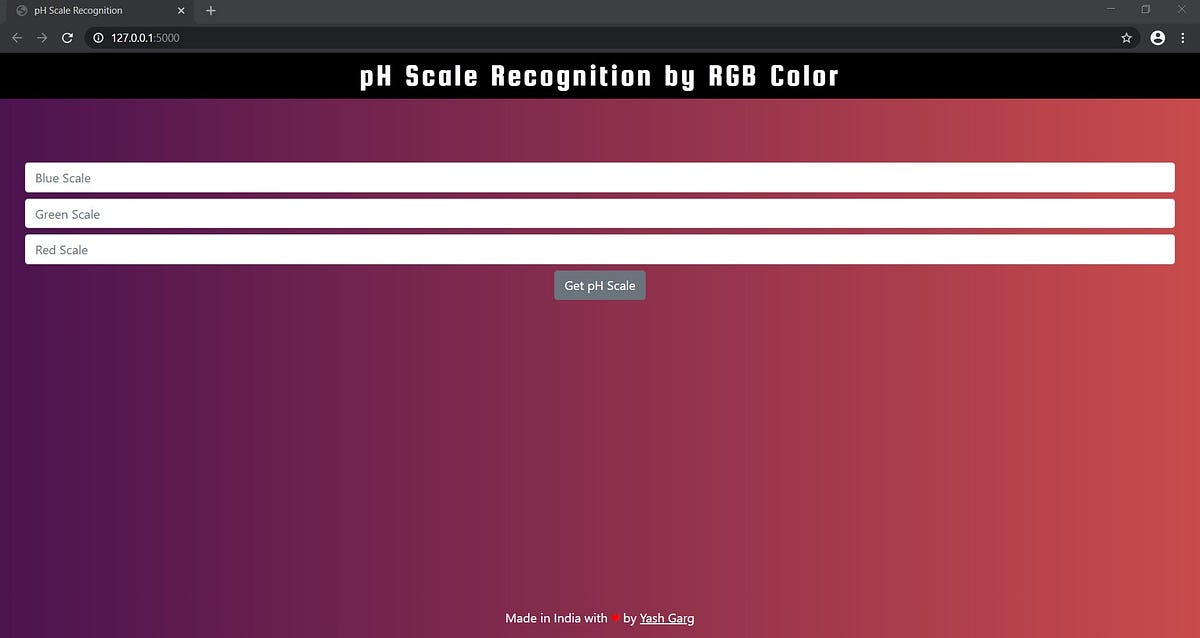# pH Scale Recognition by RGB Color Using Flask.pH scale recognition using machine learning regression model based upon the color model RGB (RED, GREEN, BLUE) and web application using Flask.

pH scale recognition using machine learning regression model based upon the color model RGB (RED, GREEN, BLUE) and web application using Flask.

Overview

A flask web application in which a user can input red, green and blue color on the scale of RGB color and gets the pH level which is between 1 and 14. It uses machine learning regression algorithms with an highest r2_score of 0.95 (Random Forest).

Domain Background

The project involves Regression using Machine Learning. The algorithms used were Multiple Linear Regression, Rigid Regression, Support Vector Machine (SVM), Decision Tree and Random Forest Regression. After the machine learning model, flask web application was bought into cyberspace.

Dataset Exploration

The dataset consists of 4 Columns:

1. Blue
2. Green
3. Red
4. Scale

The red, blue and green columns consists of color scale of their respective colors, and the scale consists of values of pH in respect to RBG.

## 15 Machine Learning and Data Science Project Ideas with Datasets

Learning is a new fun in the field of Machine Learning and Data Science. In this article, we’ll be discussing 15 machine learning and data science projects.

## Linear Regression Model for Machine Learning

An overview of the oldest supervised machine-learning algorithm, its type & shortcomings.

## Most popular Data Science and Machine Learning courses — July 2020

Most popular Data Science and Machine Learning courses — August 2020. This list was last updated in August 2020 — and will be updated regularly so as to keep it relevant

## Deploy a Machine Learning Model | Data Science | Machine Learning

Deploy a Machine Learning Model | Data Science | Machine Learning . I will train and Deploy a Machine Learning Model using Flask step by step. I will first train a model, then I will work to serve our model, and at the end I will deploy our machine learning model.

## Emotion Detection Model with Machine Learning | Data Science | Machine Learning | Python

In this article, I will take you through am Emotion Detection Model with Machine Learning. Detection of emotions means recognizing the